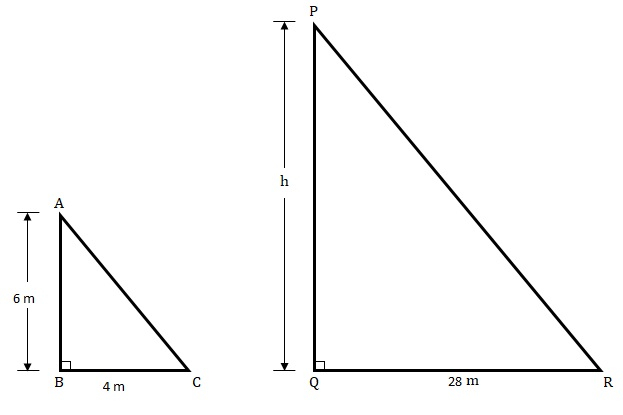# A vertical stick of length 6 m casts a shadow 4 m long on the ground and at the same time a tower casts a shadow $28 \mathrm{~m}$ long. Find the height of the tower.

Given:

A vertical stick $6\ m$ long casts a shadow $4\ m$ long.

A tower casts a shadow $28\ m$ long.

To do:

We have to find the height of the tower.

Solution:Length of the stick $= 6\ m$.

Length of the stick’s shadow $= 4\ m$.

Length of the tower’s shadow $= 28\ m$.

Let the height of the tower be $h\ m$.

In $\triangle ABC$ and $\triangle PQR$

$\angle ABC = \angle PQR = 90^o$

$\angle ACB = \angle PRQ$   (Angular elevation of Sun is same)

Therefore,

$\triangle ABC \sim\ \triangle PQR$    (By AA similarity)

This implies,

$\frac{AB}{BC} = \frac{PQ}{QR}$    (Corresponding sides are proportional)

$\frac{6}{4} = \frac{h}{28}$

$h = \frac{28\times6}{4}$

$h = 7\times6$

$h = 42\ m$

The height of the tower is $42\ m$.

Updated on: 10-Oct-2022

25 Views